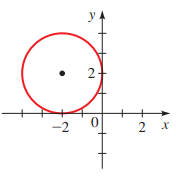# Theequation of a circle from the figure provided below,### Precalculus: Mathematics for Calcu...

6th Edition
Stewart + 5 others
Publisher: Cengage Learning
ISBN: 9780840068071### Precalculus: Mathematics for Calcu...

6th Edition
Stewart + 5 others
Publisher: Cengage Learning
ISBN: 9780840068071

#### Solutions

Chapter 1.8, Problem 101E
To determine

## To calculate: Theequation of a circle from the figure provided below,Expert Solution

Theequation of the circle is (x+2)2+(y2)2=4 .

### Explanation of Solution

Given information:

The graph of the circle is provided below,Formula used:

The standard form of the equation of the circle is (xh)2+(yk)2=r2 , where (h,k) denote the center of the circle and r denote the radius.

Distance d(A,B) between two points A=(x1,y1) and B=(x2,y2) in the Cartesian plane is denoted by d(A,B)=(x2x1)2+(y2y1)2 .

Calculation:

Consider the provided graph of the circle,It is observed that center of the circle is at (2,2) .

Since the circle passes through the point (0,2) , and center is at (2,2) therefore radius of circle is the distance between the points (0,2) and (2,2) .

Recall that the distance d(A,B) between two points A=(x1,y1) and B=(x2,y2) in the Cartesian plane is denoted by d(A,B)=(x2x1)2+(y2y1)2 .

Apply it,

d(A,B)=(20)2+(22)2=(2)2+(0)2=4=2

Recall that the standard form of the equation of the circle is (xh)2+(yk)2=r2 , where (h,k) denote the center of the circle and r denote the radius.

Compare, (h,k) and (2,2) also radius ris 2 .

Here, h=2,k=2 and r=2 .

Substitute the values in standard equation of circle,

(x(2))2+(y2)2=22(x+2)2+(y2)2=4

Thus, the equation of circle is (x+2)2+(y2)2=4 .

### Have a homework question?

Subscribe to bartleby learn! Ask subject matter experts 30 homework questions each month. Plus, you’ll have access to millions of step-by-step textbook answers!